Register for Maths, Science, English, Reasoning Olympiad Exams Register here | Book Free Demo for Live Olympiad Classes here| Check Olympiad Exam Dates here | Buy Practice Papers for IMO, IOM, HEO, IOEL etc here | Login here to participate in all India free mock test on every Saturday

# Chemical Reactions and Equations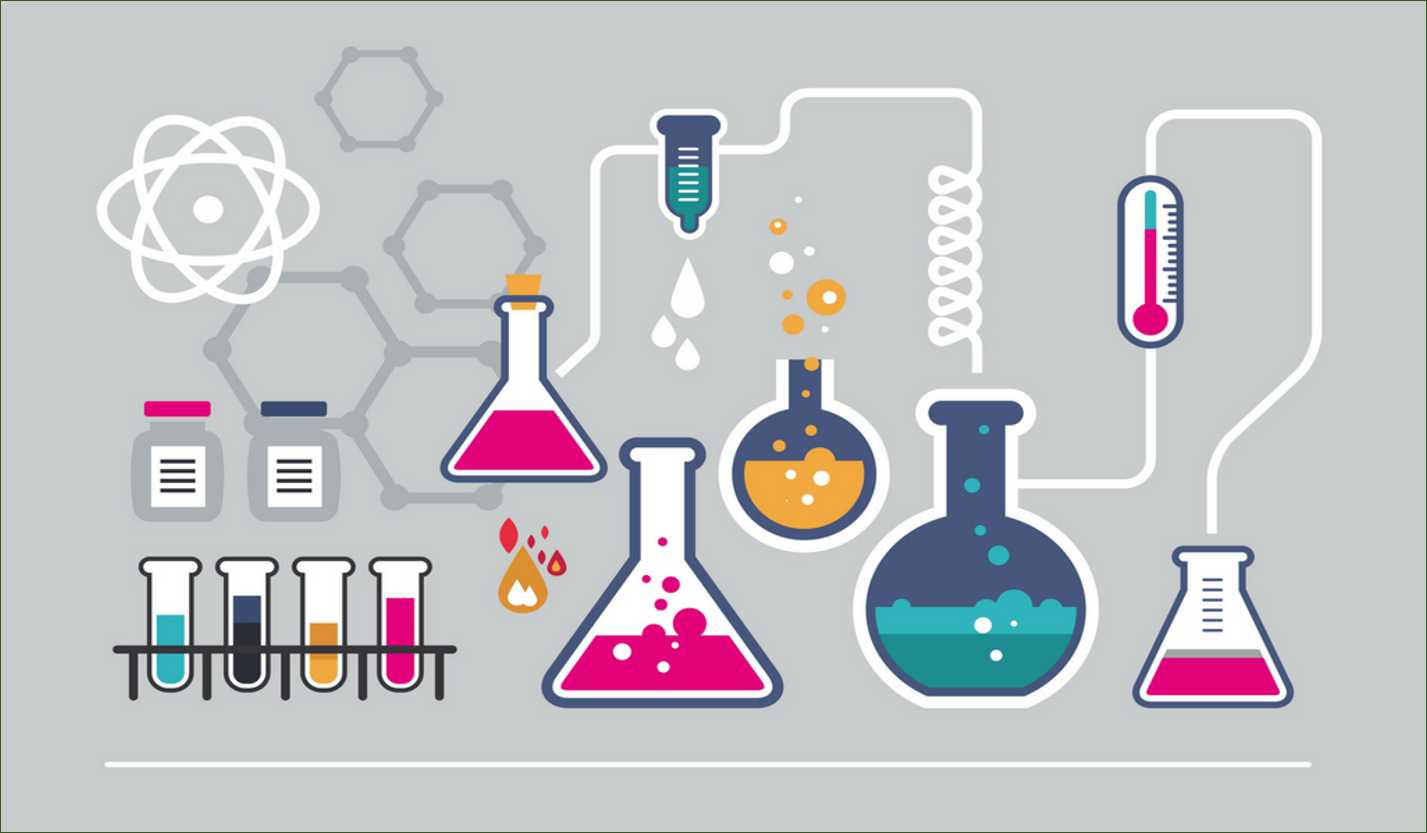In every reaction, there are certain changes which always take place in our surroundings. They can be classified as physical and chemical changes. In a physical change, no chemical reaction takes place and the body retains its properties. But in a chemical change, a chemical reaction takes place and the reactant doesn’t retain its original properties.

## Chemical Equation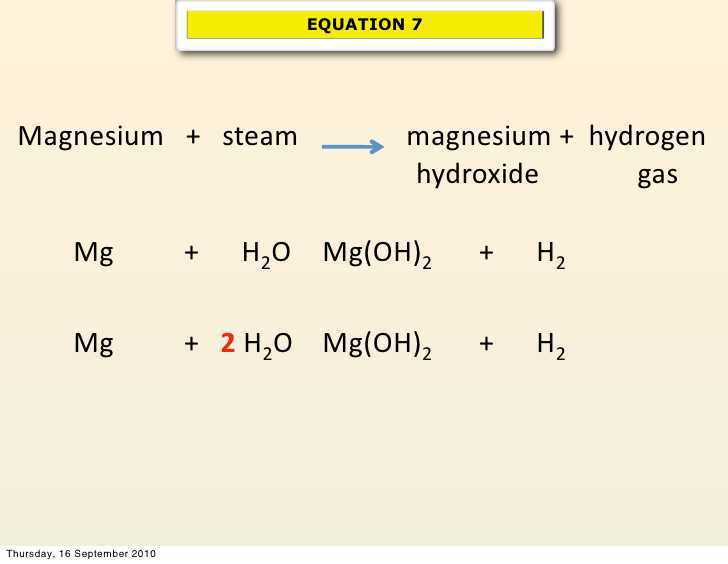When a magnesium ribbon is burnt in oxygen, it gets converted to magnesium oxide. This description of a chemical reaction in a sentence form is quite long. It can be written in a shorter form. The simplest way to do this is to write it in the form of a word-equation.

The word-equation for the above reaction would be –

Magnesium + Oxygen → Magnesium oxide

(Reactants) (Product)

The substances that undergo chemical change in this reaction, magnesium and oxygen, are the reactants. The new substance, magnesium oxide, formed during the reaction, is the product.

A word-equation shows change of reactants to products through an arrow placed between them. The reactants are written on the left-hand side (LHS) with a plus sign (+) between them. Similarly, products are written on the right-hand side (RHS) with a plus sign (+) between them. The arrowhead points towards the products and shows the direction of the reaction.

## Balanced Chemical Equations

Mass can neither be created nor destroyed in a chemical reaction. That is, the total mass of the elements present in the products of a chemical reaction has to be equal to the total mass of the elements present in the reactants. In other words, the number of atoms of each element remains the same, before and after a chemical reaction.

### Steps to balance the balanced equation:

Step 1: To balance a chemical equation, first draw boxes around each formula. Do not change anything inside the boxes while balancing the equation.

Step 2: List the number of atoms of different elements present in the unbalanced equation.

Step 3: It is often convenient to start balancing with the compound that contains the maximum number of atoms. It may be a reactant or a product. In that compound, select the element which has the maximum number of atoms. Using these criteria, we select Fe3O4 and the element oxygen in it. There are four oxygen atoms on the RHS and only one on the LHS.

Step 4: Fe and H atoms are still not balanced. Pick any of these elements to proceed further. Let us balance hydrogen atoms in the partly balanced equation. To equalize the number of H atoms, make the number of molecules of hydrogen as four on the RHS.

Step 5: Examine the above equation and pick up the third element which is not balanced. You find that only one element is left to be balanced, that is, iron.

Step 6: Finally, to check the correctness of the balanced equation, we count atoms of each element on both sides of the equation.

## Types Of Chemical Equations

1. Combination Reaction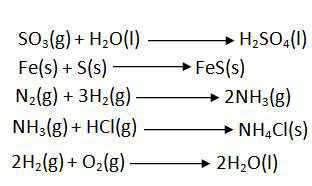1. Decomposition Reaction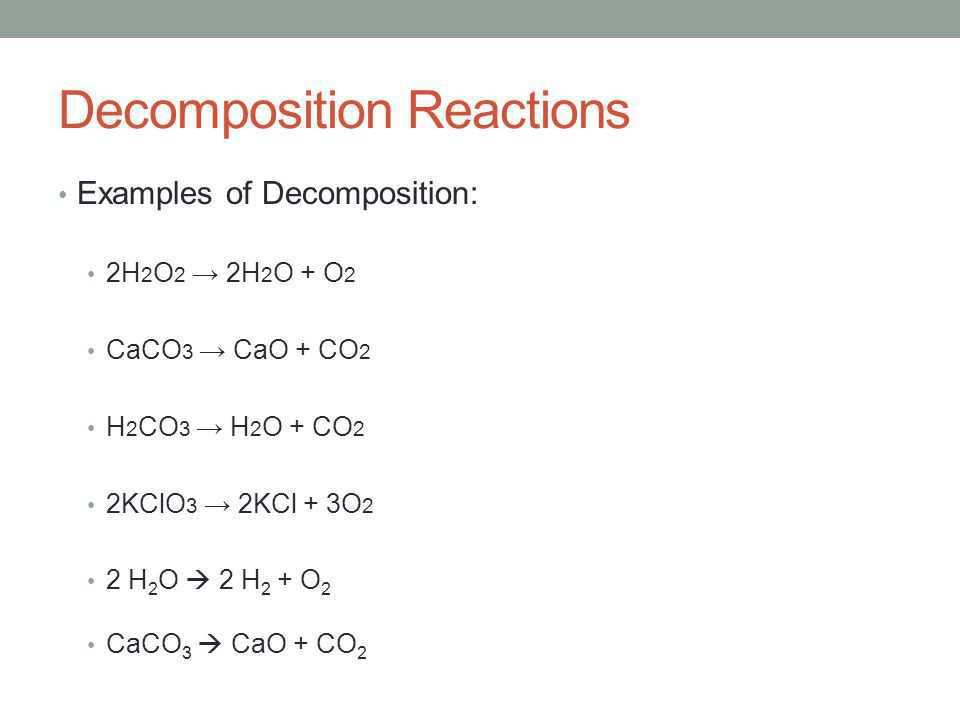1. Displacement Reaction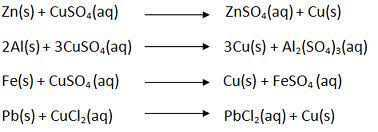1. Double displacement reaction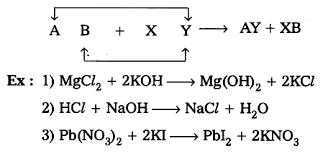## Recap

• A complete chemical equation represents the reactants, products and their physical states symbolically.
• A chemical equation is balanced so that the numbers of atoms of each type involved in a chemical reaction are the same on the reactant and product sides of the equation. Equations must always be balanced.
• In a combination reaction two or more substances combine to form a new single substance.
• Decomposition reactions are opposite to combination reactions. In a decomposition reaction, a single substance decomposes to give two or more substances.
• Reactions in which heat is given out along with the products are called exothermic reactions.
• Reactions in which energy is absorbed are known as endothermic reactions.
• When an element displaces another element from its compound, a displacement reaction occurs.
• Two different atoms or groups of atoms (ions) are exchanged in double displacement reactions.
• Precipitation reactions produce insoluble salts.
• Reactions also involve the gain or loss of oxygen or hydrogen by substances.
• Oxidation is the gain of oxygen or loss of hydrogen. Reduction is the loss of oxygen or gain of hydrogen.## Quiz for Chemical Reactions and Equations

 Q.1 The symbol H stands for ____ of hydrogen. a) one atom b) one molecule c) one ion d) two atoms
 Q.2 The correct formula for nitrogen dioxide is a) NO b) N2O c) NO2 d) N2O5
 Q.3 The correct formula for ammonium sulphate is _____. a) NH4SO4 b) (NH4)2SO4 c) (NH3)2SO4 d) (NH4)2(SO4)2
 Q.4 Which of the following is an incorrect formula? a) NaCl2 b) BaSO4 c) H2CO3 d) P2O5
 Q.5 Which of the following changes indicates that a chemical reaction has taken place? a) Change in volume b) Change in odour c) Change in temperature d) Cannot be predicted .
 Q.6 Which of the following changes cannot confirm the occurrence of the chemical reaction? a) Change in odour b) Change in volume c) Evolution of gas d) Change in temperature
 Q.7 The value of x and y after balancing the given equation will come to be 3 Fe + xH2O → Fe3O4 + yH2 _________. a) x=2 , y=4 b) x=3 , y=2 c) x=4 , y=2 d) x=4 , y=4
 Q.8 The value of a and b after balancing the given equation will come to be an Mg + O2 → b MgO ______. a) a= 1 , b= 2 b) a=2 , b=2 c) a=2, b=1 d) a=1 , b=3
 Q.9 Ankita in her science class was asked by her teacher - " Write the chemical reaction when aluminum sulphate reacts with barium chloride to produce aluminium chloride and barium sulphate ." Since Ankita is little weak in balancing help her …It will make you practice too !! Here are the three equation choose the correct one - i- Al2(SO4)3 + 3BaCl2 → 2AlCl3 + 3BaSO4 ii- 2Al2(SO4)3 + 3BaCl2 → 2AlCl3 + 3BaSO4 iii- Al2(SO4)3 + 5BaCl2 → 2AlCl3 + 3BaSO4 a) ii b) i c) iii d) All are correct
 Q.10 Which of the following is not a type of chemical reactions? a) Aromatic b) Decomposition c) Redox d) Displacement